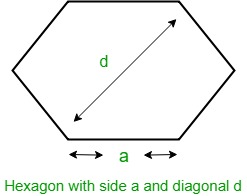Open in App
Not now

# Area of hexagon with given diagonal length

• Last Updated : 27 Aug, 2022

You are given the length of the diagonal of a hexagon, d. Your task is to find the area of that hexagon.
Examples:

Input :   5
Output :   Area of Hexagon: 16.238

Input : 10
Output : Area of Hexagon: 64.9519

Hexagon
Hexagon is a regular polygon having six equal sides and all equal angles. The interior angles of Hexagon are of 120 degrees each and the sum of all angles of a Hexagon is 720 degrees.Let d be the diagonal of Hexagon, then the formula to find the area of Hexagon given by
Area =How does above formula work?
We know that area of hexagon with side length a = (3 √3(a)2 ) / 2. Since all sides are of same size and angle is 120 degree, d = 2a or a = d/2. After putting this value, we get area as (3 √3(d)2 ) / 8.
Below is the implementation .

## C++

 // C++ program to find the area of Hexagon with given diagonal #include  using namespace std;   // Function to calculate area float hexagonArea(float d) {     // Formula to find area     return (3 * sqrt(3) * pow(d, 2)) / 8; }   // Main int main() {     float d = 10;     cout << "Area of hexagon: " << hexagonArea(d);     return 0; }

## Java

 // Java program to find the area of  // Hexagon with given diagonal  import java.lang.Math;    public class GfG {       // Function to calculate area      public static float hexagonArea(float d)      {          // Formula to find area          return (float)((3 * Math.sqrt(3) * d * d) / 8);      }        public static void main(String []args) {         float d = 10;         System.out.println("Area of hexagon: " + hexagonArea(d));     } }   // This code is contributed by Rituraj Jain

## Python3

 # Python3 program to find the area  # of Hexagon with given diagonal  from math import sqrt   # Function to calculate area  def hexagonArea(d) :       # Formula to find area      return (3 * sqrt(3) * pow(d, 2)) / 8   # Driver code if __name__ == "__main__" :        d = 10     print("Area of hexagon:",             round(hexagonArea(d), 3))   # This code is contributed by Ryuga

## C#

 // C# program to find the area of  // Hexagon with given diagonal    using System;   public class GFG{           // Function to calculate area      public static float hexagonArea(float d)      {          // Formula to find area          return (float)((3 * Math.Sqrt(3) * d * d) / 8);      }      //Code driven     static public void Main (){             float d = 10;         Console.WriteLine("Area of hexagon: " + hexagonArea(d));     } //This code is contributed by Tushil.     }

## PHP

 

## Javascript

 

Output:

Area of Hexagon:  64.952

Time Complexity: O(1)

Auxiliary Space: O(1), since no extra space has been taken.

My Personal Notes arrow_drop_up
Related Articles